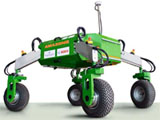## Biological Systems Engineering## Biological Systems Engineering: Papers and Publications

2001

#### Citation

Transactions of the ASAE, Vol. 44(6): 1625–1633

Copyright 2001 American Society of Agricultural Engineers

#### Abstract

In a previous study, we demonstrated that fuzzy evapotranspiration (ET) models can achieve accurate estimation of daily ET comparable to the FAO Penman–Monteith equation, and showed the advantages of the fuzzy approach over other methods. The estimation accuracy of the fuzzy models, however, depended on the shape of the membership functions and the control rules built by trial–and–error methods. This paper shows how the trial and error drawback is eliminated with the application of a fuzzy–neural system, which combines the advantages of fuzzy logic (FL) and artificial neural networks (ANN). The strategy consisted of fusing the FL and ANN on a conceptual and structural basis. The neural component provided supervised learning capabilities for optimizing the membership functions and extracting fuzzy rules from a set of input–output examples selected to cover the data hyperspace of the sites evaluated. The model input parameters were solar irradiance, relative humidity, wind speed, and air temperature difference. The optimized model was applied to estimate reference ET using independent climatic data from the sites, and the estimates were compared with direct ET measurements from grass–covered lysimeters and estimations with the FAO Penman–Monteith equation. The model–estimated ET vs. lysimeter–measured ET gave a coefficient of determination (r2) value of 0.88 and a standard error of the estimate (Syx) of 0.48 mm d–1. For the same set of independent data, the FAO Penman–Monteith–estimated ET vs. lysimeter–measured ET gave an r2 value of 0.85 and an Syx value of 0.56 mm d–1. These results show that the optimized fuzzy–neural–model is reasonably accurate, and is comparable to the FAO Penman–Monteith equation. This approach can provide an easy and efficient means of tuning fuzzy ET models.

COinS